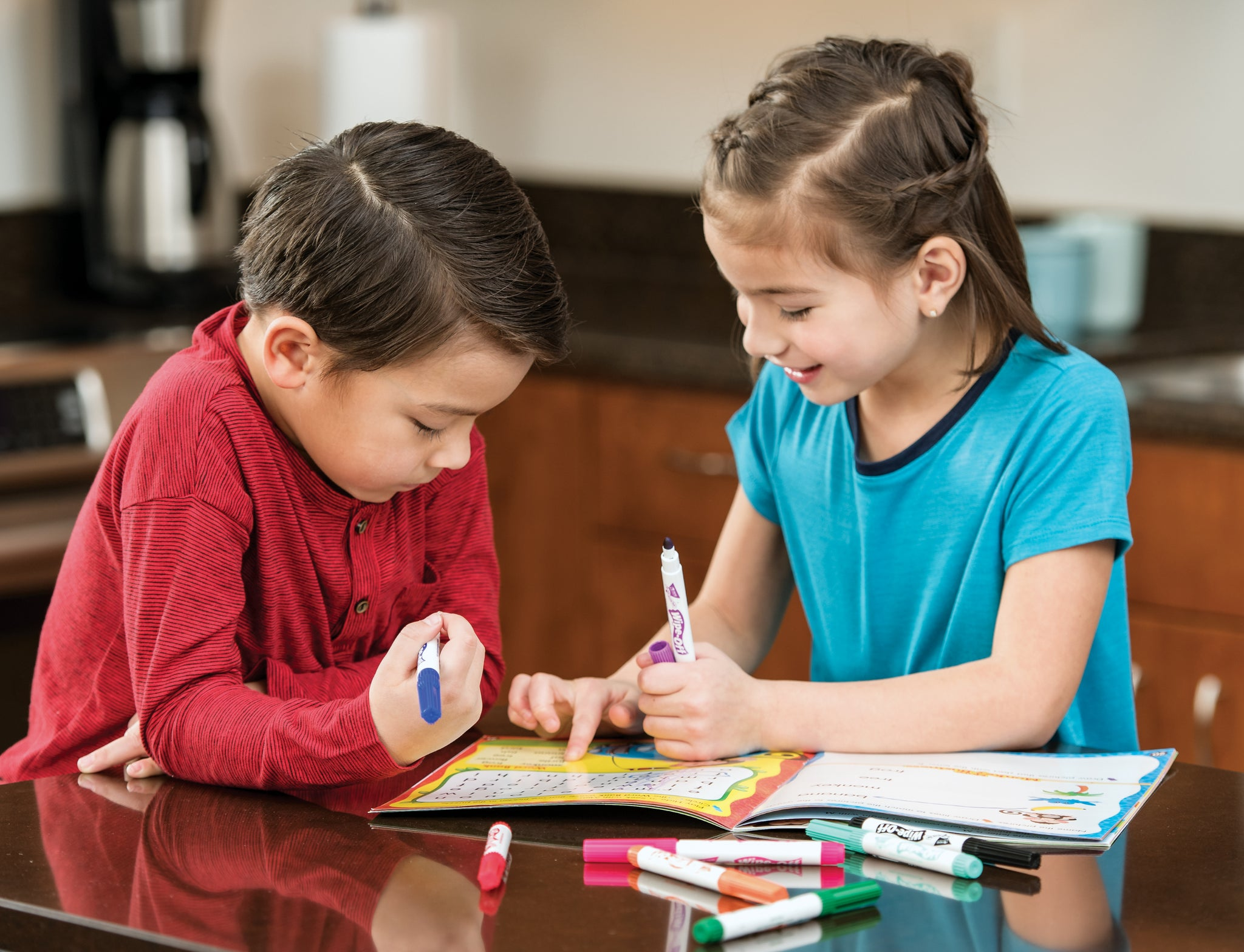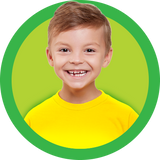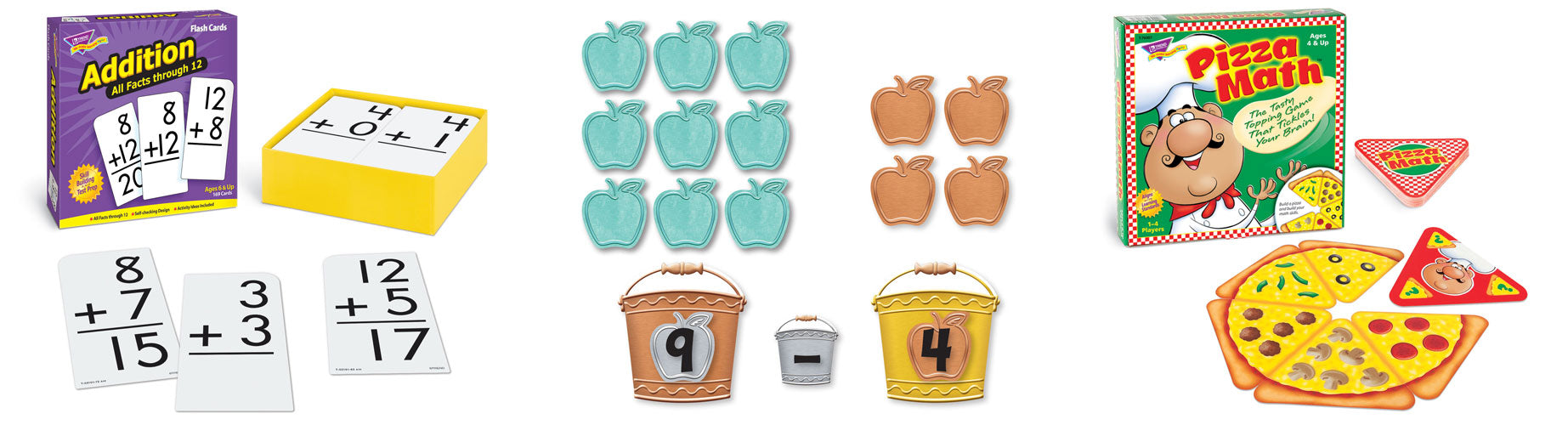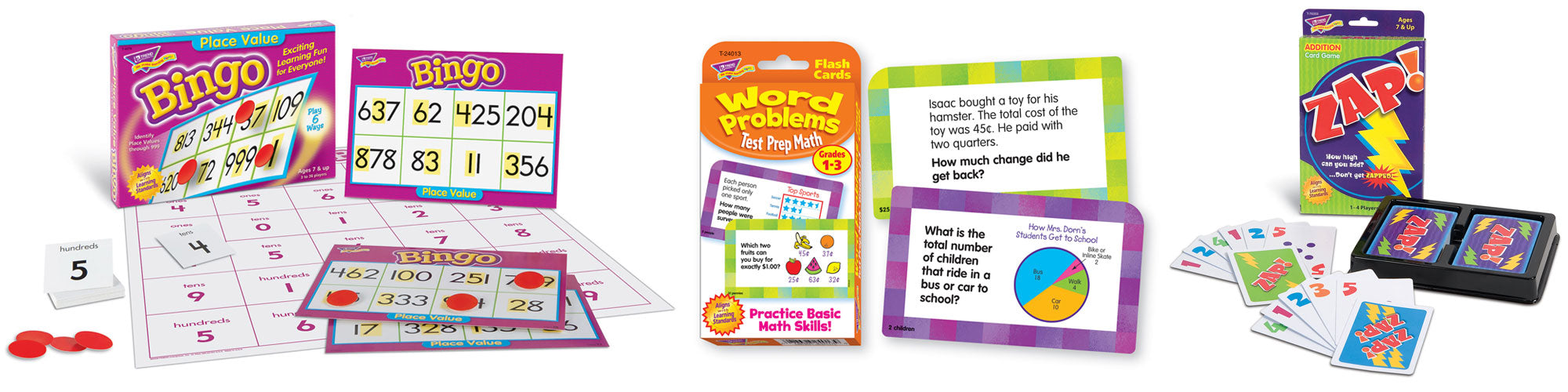# How to Improve Math Skills for Kindergarten through 5th GradeTelling time, counting money, taking attendance, and measuring ingredients are only some of the many benefits of learning math. Kids may not realize it, but math is an important part of their daily lives. Building critical, lifelong math skills begins at an early age and takes time to master.

It’s important for parents and educators to keep kids engaged and interested in learning math concepts from the start. Helping children build a core understanding of the basics, like addition and subtraction, is crucial for learning more complicated concepts, like multiplication, fractions, geometric measurements, and so on.

How do you give children the knowledge they need and keep their interest? Make learning math FUN! When learning is fun, students are less likely to be intimidated and overwhelmed, and are more likely to take interest in the subject, push through challenges, retain information, and succeed.

Read on for helpful guidelines for skill development for each grade level, K-5. Plus, see our tips and ideas for how to make math fun while improving skills and reinforcing concepts at home.

# General Math Benchmarks for Grades K-5

The following lists show important skills and concepts to learn for each grade level, adopted from ParentToolkit.com. Remember, each child learns at his or her own pace. We recommend parents and teachers work together to determine individual readiness.

###Kindergarten Math Skills:

• Understanding and recognizing numbers (i.e. number names and numerals)
• Reading and writing numbers 1-20 and counting to 100
• Adding and subtracting up to 10 and understanding basic principles of addition (e.g. “adding to,” “putting together”) and subtraction (e.g. “taking from,” “taking apart”)
• Understanding basic place value with numbers 11-19 (e.g. 17 = 1 ten and 7 ones)
• Counting and comparing objects and their attributes (e.g. circle, square, cube, etc.)

###1st Grade Math Skills:

•  Reading and writing numbers 20-120 and counting forward between 1-120
• Solving addition and subtraction problems that involve numbers 1-20 (e.g. number sentences and word problems)
• Understanding math symbols (e.g. minus sign is for subtraction, etc.) and place value for numbers of one or two digits and categories of tens (e.g. 50 = 5 tens or 50 ones)
• Comparing numbers using math symbols (i.e. >, <, =)
• Dividing shapes like circles and squares into halves and quarters
• Measuring and organizing objects based on different measurements or attributes (e.g. length, size, color, etc.)

###2nd Grade Math Skills:

• Reading and writing numbers up to 1000, and counting up to 1000
• Understanding and counting odd and even numbers
• Further developing addition and subtraction skills
• Understanding hundreds place value and the relationship between ones, tens, and hundreds
• Adding and subtracting with numbers 1-1000
• Solving more difficult problems, including one-step and two-step problems, problems with large-digit numbers, and word problems involving money
• Telling, reading, and writing time
• Representing measurements and data using picture graphs and bar graphs

###3rd Grade Math Skills:

• Understanding what multiplication and division means and their relationship with the concepts of addition
• Understanding the relationship between multiplication and division (e.g. 8 x 4 = 32 is the same as 32 ÷ 4 = 8 and 32 ÷ 8 = 4)
• Knowing the times table
• Solving word problems using multiplication and division of numbers 1-100 and word problems involving mass and volume
• Understanding fractions as numbers and units
• Comparing fractions using numerators and denominators
• Using graphs to interpret data

###4th Grade Math Skills:

• Developing knowledge and application of fractions (e.g. ordering fractions, adding and subtracting fractions, working with denominators and numerators, working with mixed numbers, multiplying whole numbers by fractions, etc.)
• Understanding the relationships between fractions and decimals
• Solving more complicated, multi-step word problems involving addition, subtraction, multiplication, and division with multi-digit numbers and/or units of time, money, mass, weight, volume, and distance/length
• Understanding the geometric line of symmetry and measurements like perimeter, lines, and angles

###5th Grade Math Skills:

• Understanding place value in relation to decimals up to the thousandths place and comparing decimals using mathematical symbols (i.e. <, >, =)
• Understanding exponents and the different ways to represent them (e.g. 52 = 5 x 5 and 53 = five cubed or five to the third power)
• Adding, subtracting, multiplying, and dividing fractions with other fractions and whole numbers and finding the common denominators
• Solving word problems involving addition, subtraction, multiplication, and division of fractions
• Converting units within systems of measurement (e.g. liters to milliliters, etc.)
• Using geometric formulas to determine the volume of three-dimensional objects# Fun Math Help for Kids

Learning math can be very fun, exciting, and rewarding for kids with the right approach. Use these tips to keep math learning approachable and FUN outside the classroom.

## Make math real and relatable!

Incorporate real-world math into your family’s daily routine. Applying math in your day-to-day is a great way to “secretly” practice concepts kids are learning in school, give them an understanding of the real-world significance and benefits of math skills, plus its a great opportunity for kids to proudly show what they know!

We’ve listed a few ideas below to get you started. Refer to your child’s current curriculum and the benchmarks above to expand our ideas to best support his or her development. Ideas include:
• Invite your kindergartener to set the table by counting out the number of plates

## Be involved and offer help.

While it can be good practice to challenge learners, it's also important to offer them support before they become frustrated. Frustrated learners are more likely to give up. Talk through the problems and find solutions together!
• Keep an open dialogue with your child so they feel comfortable asking for help.
• Check over math homework and practice worksheets for understanding and mastery to identify potential issues and to see (and celebrate!) areas of improvement.
• It’s OK if you’re not an expert at math. Learning together is a great way to show kids perseverance and give them a learning process to model.
• Provide additional problems and math exercises to help kids solidify their newly acquired skills … remember to always end your sessions with a success!

## Support learning with FUN activities kids enjoy!

It’s great to associate learning concepts with positive experiences, especially when topics may be initially challenging or frustrating.

Need FUN ideas for math activities? Try a variety of active learning options, like math brain teasers for kids, math puzzles for kids, and math activities, card games, and board games for kids.

# Math Help: Our Favorites

We’ve listed some of our favorite math tools and activity ideas below. These are great for improving kids’ math skills and reinforcing learning in playful, exciting ways.

## Kindergarten: